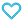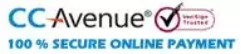# Plane Trigonometry Part-1

95.00

Plane trigonometry -part 1 is a valuable study resource of school students as well as students of Junior Honours university classes. Important formulae in trigonometry are provided in the book for clear understanding of concepts with a series of questions for practice. Also, Logarithm tables are given at the end of the book.

896 in stock

Qty:Add to WishlistTable of Content:-
• 1. Measurement of Angles; Sexagesimal and Centesimal Measure Circular, or Radian, Measure
• 2. Trigonometrical Ratios for Angles Less than a Right Angle Values of angles of 45°, 30°, 60°, 0° and 90°
• 3. Simple Problems in Heights and Distances
• 4. Applications of Algebraic Signs to Trigonometry  Tracing the Changes in the Ratios
• 5. Trigonometrical Functions of Angles of any Size and Sign  Ratios for –q, 90° – q, 90° + q, ......
• 6. General Expressions for all Angles having a Given Trigonometrical Ratio
• 7. Trigonometrical Ratios of the Sum and Difference  of Two Angles Product Formulae
• 8. Trigonometrical Ratios of Multiple and Submultiple  Angles Explanation of ambiguities Angles of 18°, 36° and 9°
• 9. Identities and Trigonometrical Equations
• 10. Logarithms  Table of Logarithms
• 11. The Principle of Proportional Parts
• 12. Relations Between the Sides and
•  The Trigonometrical Ratios of the Angles of
•  any Triangle
• 13. Solution of Triangles
•  Given Two Sides and the Included Angle
•  Ambiguous Case
• 14. Heights and Distances
• 15. Properties of a Triangle
•  The Circles Connected with a given Triangle
•  Orthocentre and Pedal Triangle
•  Centroid and Medians
• 16. Quadrilaterals and Regular Polygons
• 17. Trigonometrical Ratios of Small Angles.
•  sin Ø<Ø< tan Ø
•  Area of a Circle.
•  Dip of the Horizon.
• 18. Inverse Circular Functions
• 19. Summation of Some Simple Trigonometrical Series
• 20. Elimination
• 21. Projections
•  Miscellaneous Examples